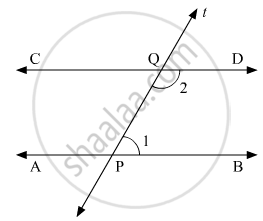# If Two Interior Angles on the Same Side of a Transversal Intersecting Two Parallel Lines Are in the Ratio 2:3, Then the Measure of the Larger Angle is - Mathematics

MCQ

If two interior angles on the same side of a transversal intersecting two parallel lines are in the ratio 2:3, then the measure of the larger angle is

•  54°

•  120°

•  108°

•  136°

#### Solution

Let us draw the following figure:Here AB || CD with t as a transversal.

Also, ∠1and ∠2are the two angles on the same side of the transversal.

It is given that

∠1:∠2 = 2:3

Therefore, let

∠1 = 2x

and ∠2 = 3x

We also, know that, if a transversal intersects two parallel lines, then each pair of consecutive interior angles are supplementary.

Therefore,

∠1 + ∠2 = 180°

On substituting ∠1 =2x  and ∠2 = 3x in equation above, we get:

2x +3x = 180°

5x = 180°

x = (180°) /5

x = 36°

Clearly, 3x >2x

Therefore,

∠2 > ∠1

Also,

∠2 = 3x

∠2 = 3(36°)

∠2 = 108°

Concept: Parallel Lines and a Transversal
Is there an error in this question or solution?
Chapter 10: Lines and Angles - Exercise 10.6 [Page 53]

#### APPEARS IN

RD Sharma Mathematics for Class 9
Chapter 10 Lines and Angles
Exercise 10.6 | Q 10 | Page 53

Share